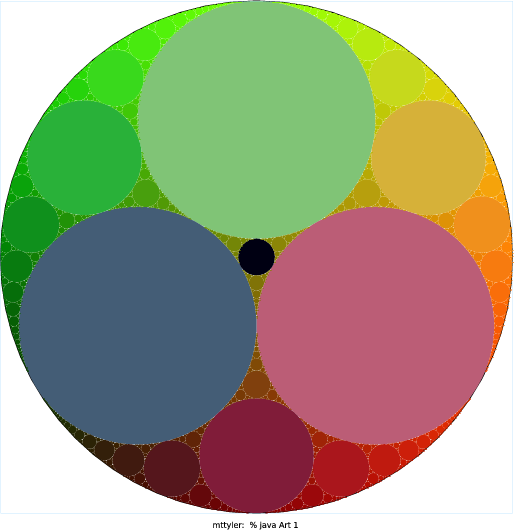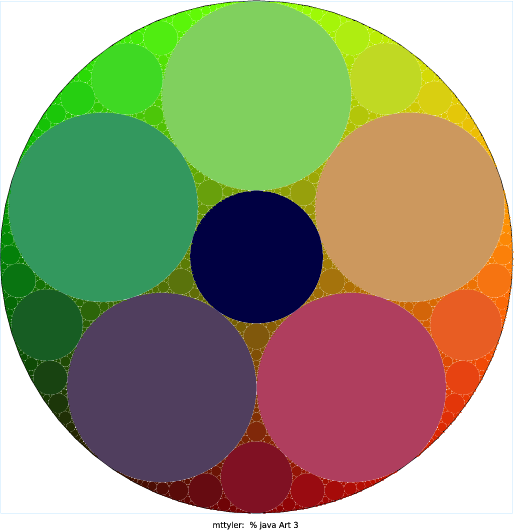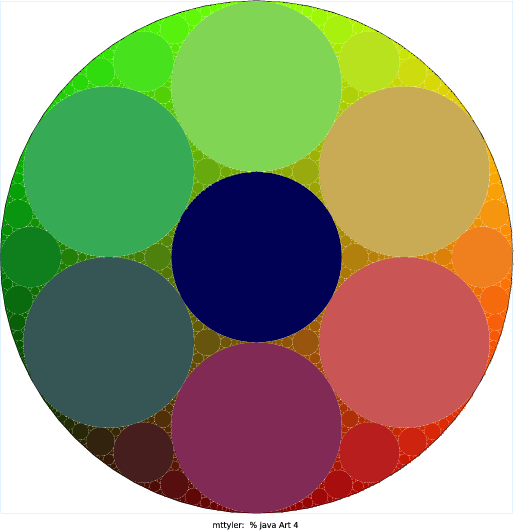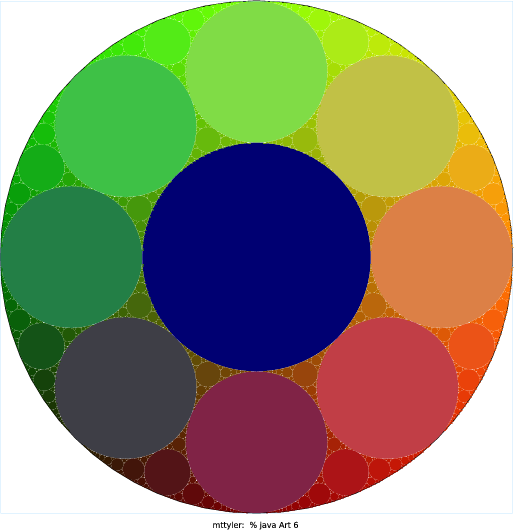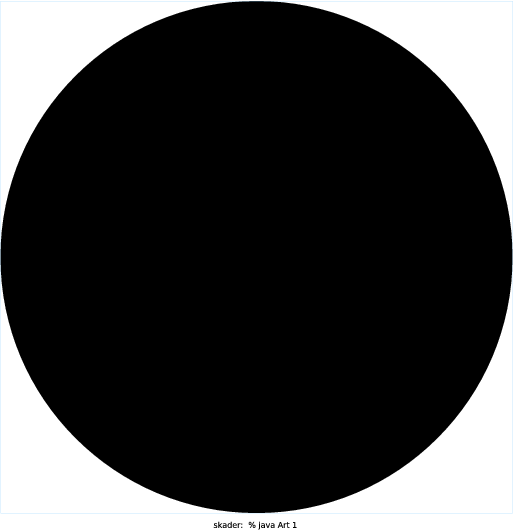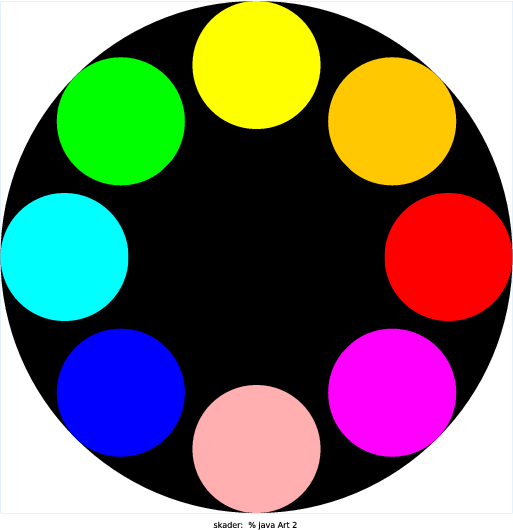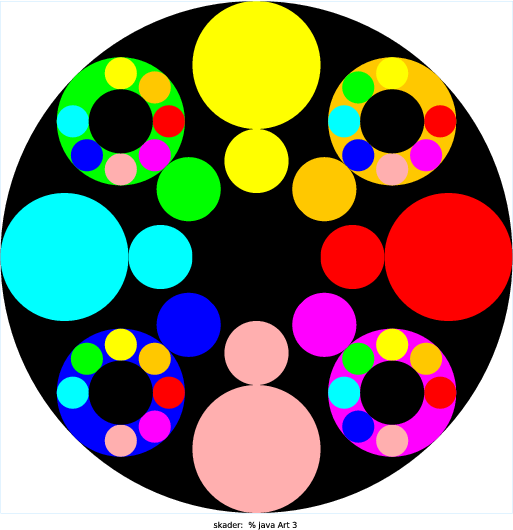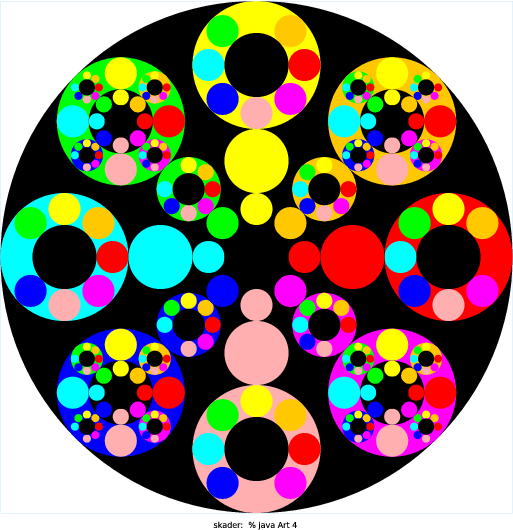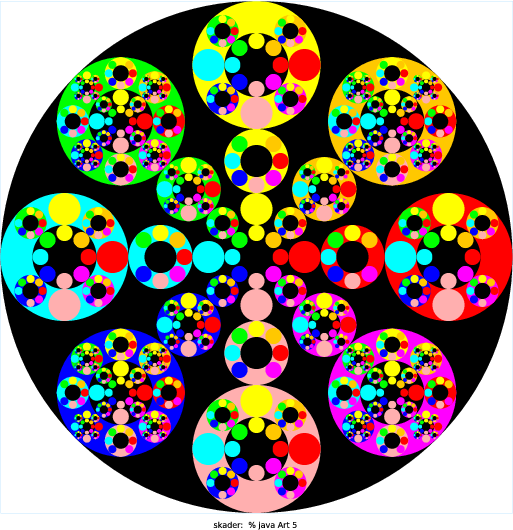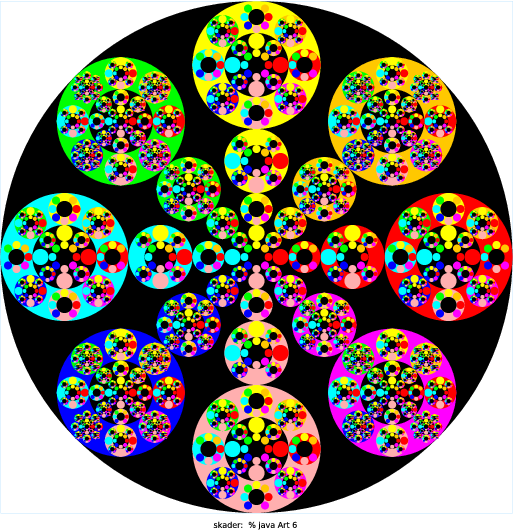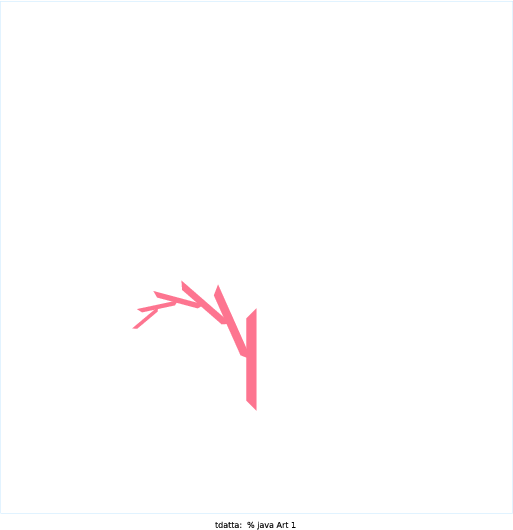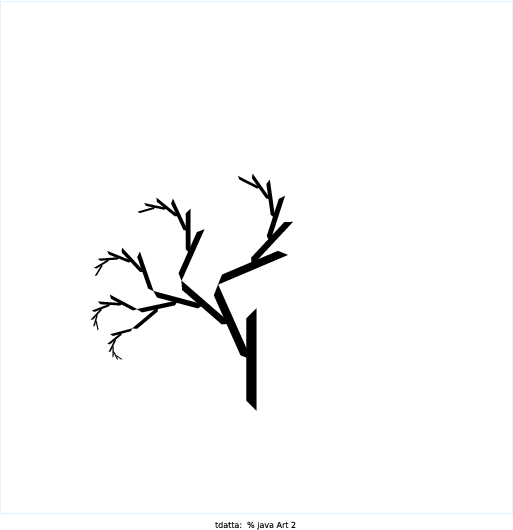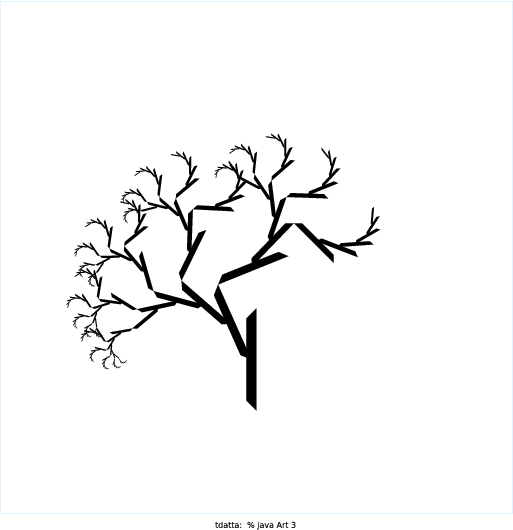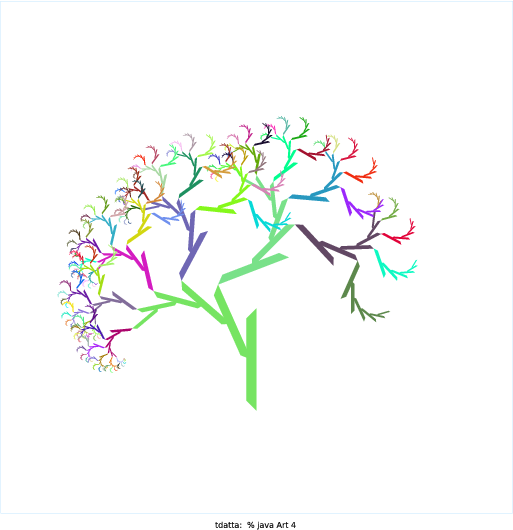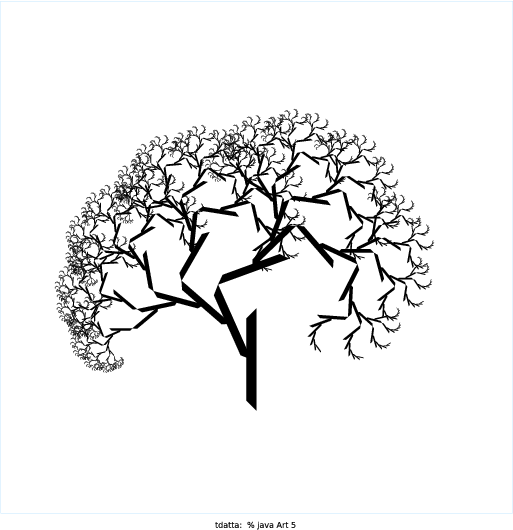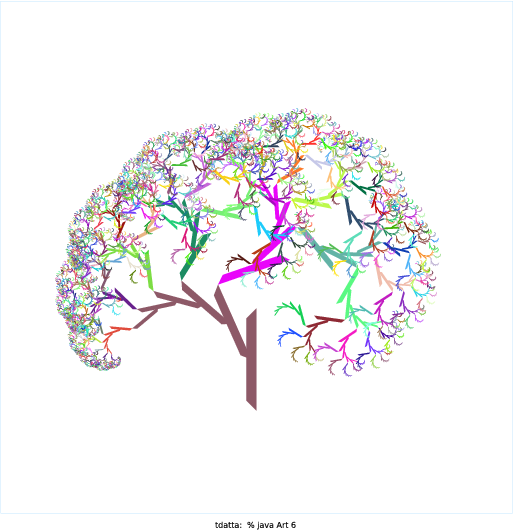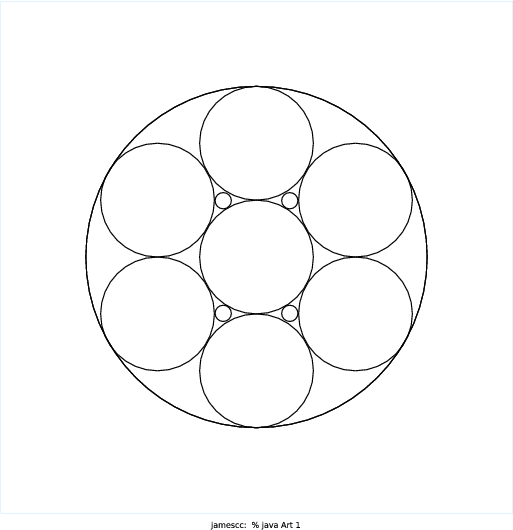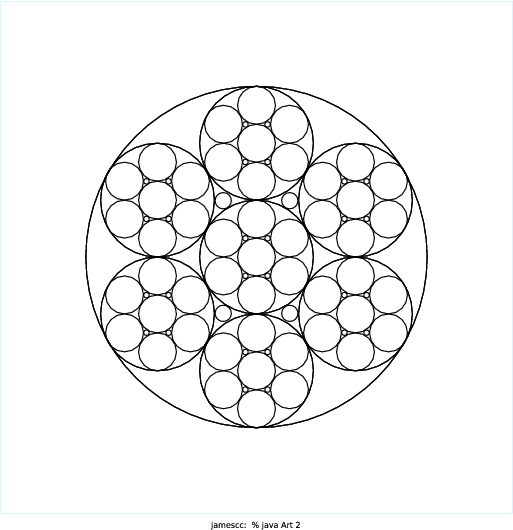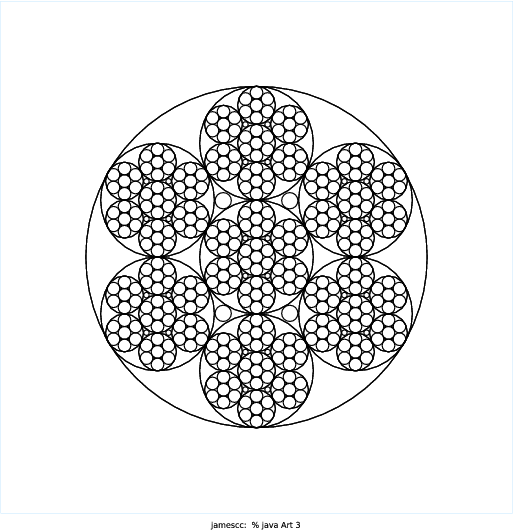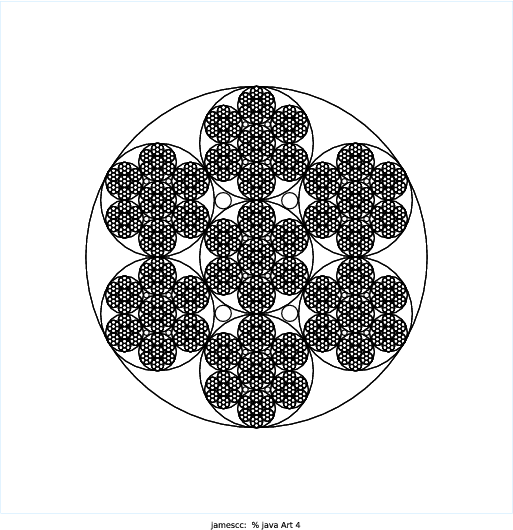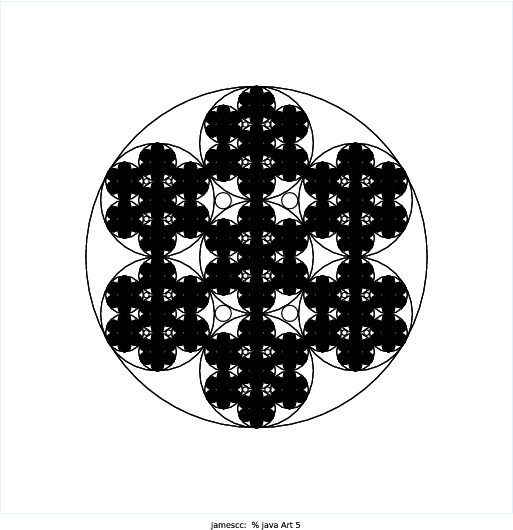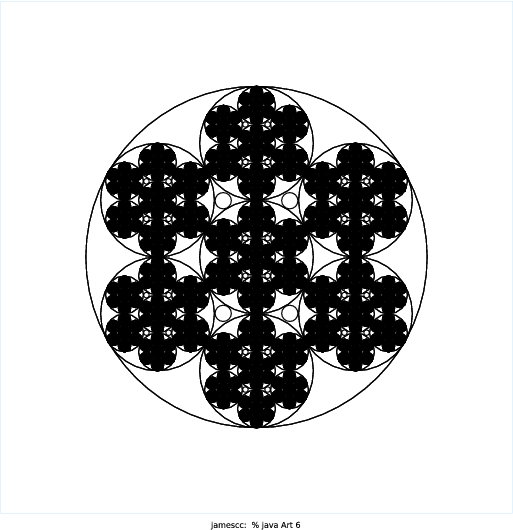# COS 126 Recursive Graphics Submissions, Fall 2015

NAME AND DESCRIPTION N = 1 N = 2 N = 3 N = 4 N = 5 N = 6
Jack Magill

I created a 2D visualization of a Menger Sponge. The Menger Sponge beings as a cube with side length, s. Then it is split up into 27 cubes each with side length s/3. The 'middle' cubes are removed, a total of 7 (one on each face and the center one) of them. The process is then repeated on the remaining 20 cubes, each being split into 27 cubes with side length s/9, etc. After infinite steps, the cube has zero volume but infinite surface area, which is a cool property. I used a basic 2D projection of the cube, which complicated the calculations but was enjoyably challenging. There are two methods, one which produces the fractal on the top face, and the second which deals with the left and right faces (which are y-axis symmetrical). Each call to the 'face' methods has 8 recursive calls within.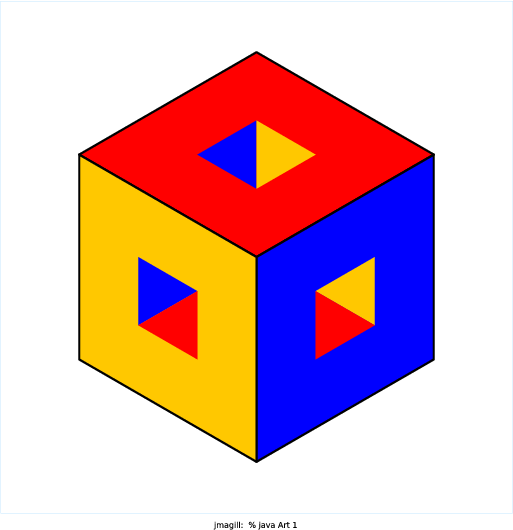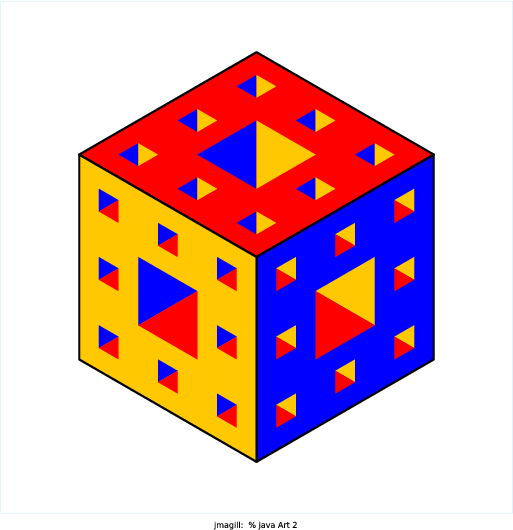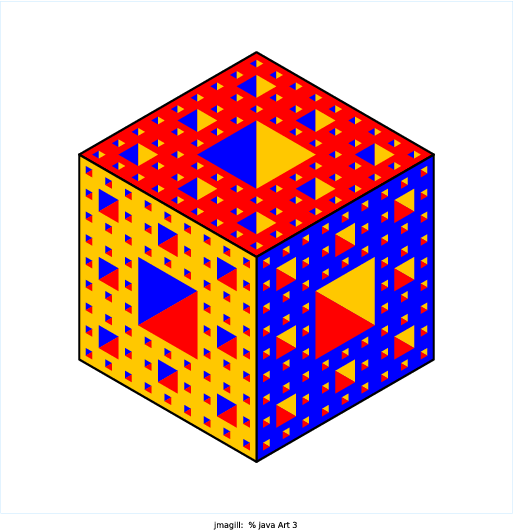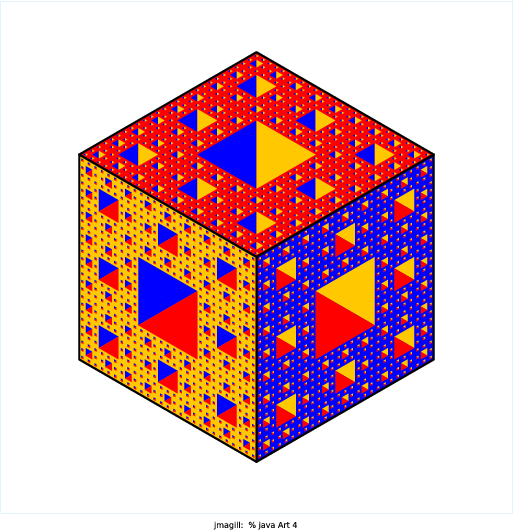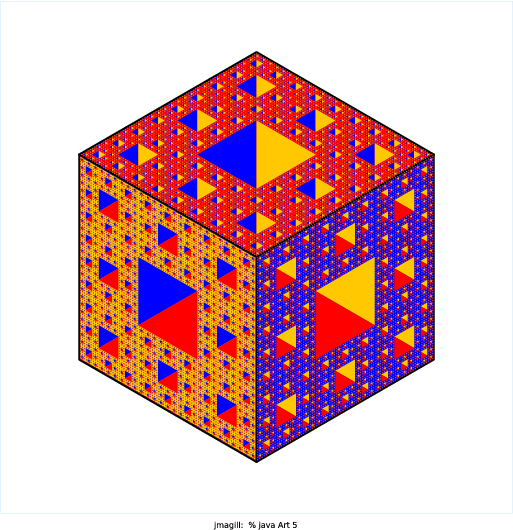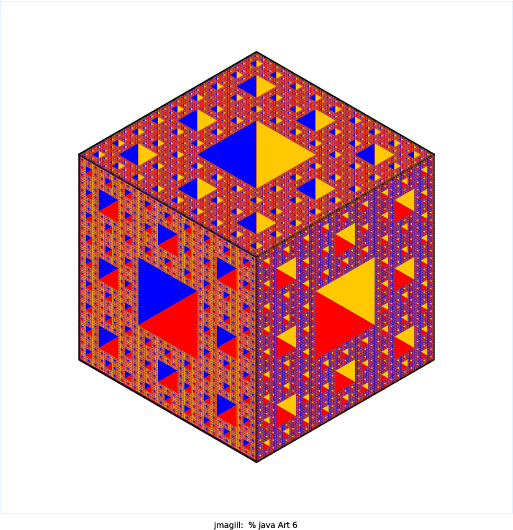Gerry Wan

I was inspired by both the Menger Sponge and the AOPS logo. I wanted to create something that looked 3D, so I played with different colorings of hexagons to create the illusion of cubes. After figuring out the method for creating the cubes, I used mutual recursion as well as branches that had a different number of recursive calls per level to extrude and hollow out the corners of each cube. The end result was a cool pattern of differently oriented cubes.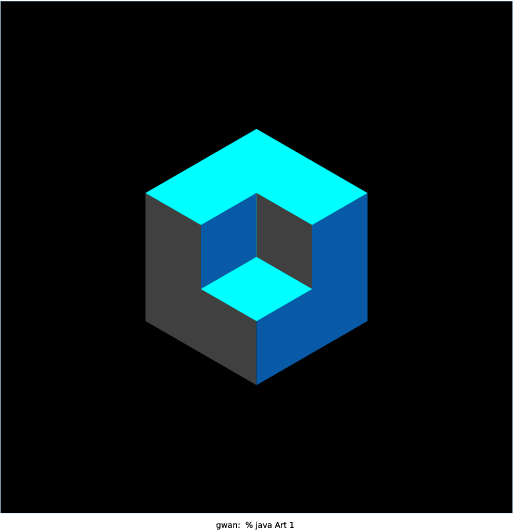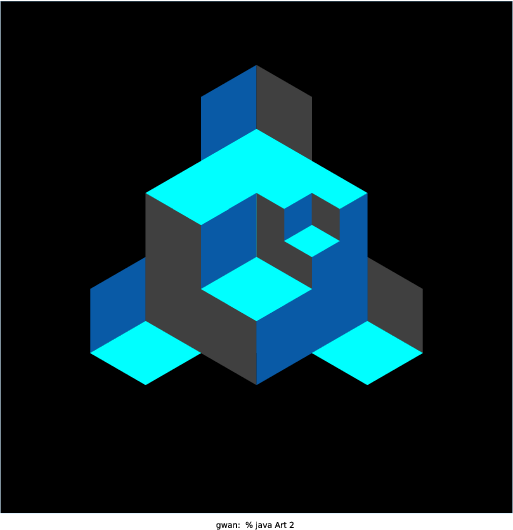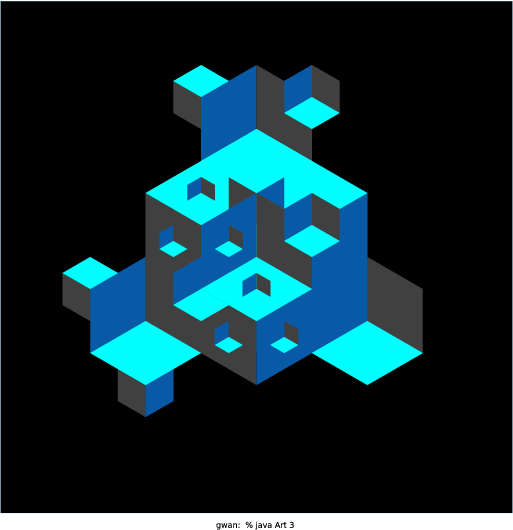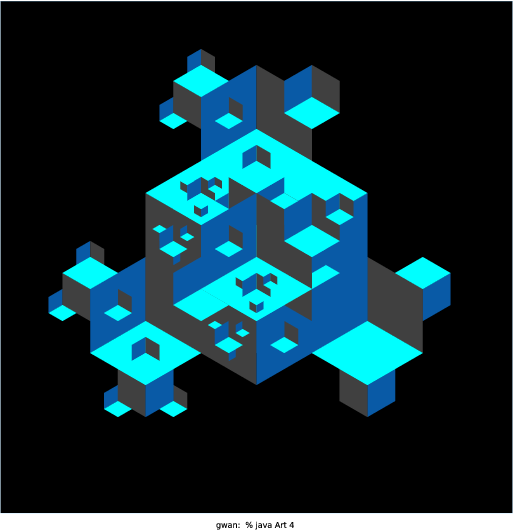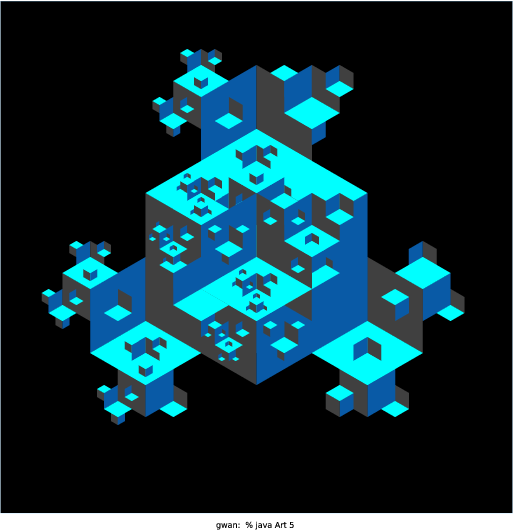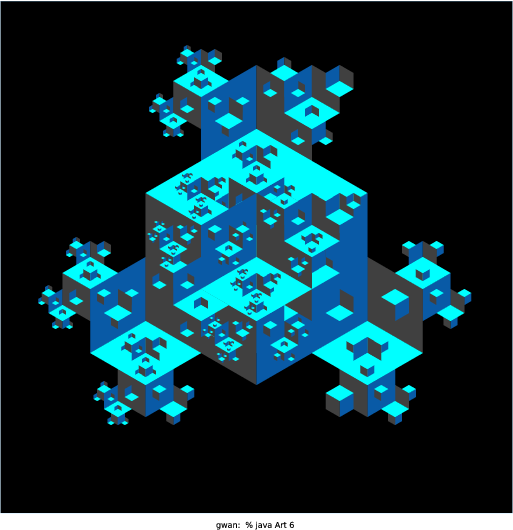Alice Tao

My version of Art.java draws a Mondrian-esque square with red, blue, white, or yellow blocks inside the main square. Mondrian does use Golden Rectangle in his paintings and at first I tried using the equation in this article: http://mathworld.wolfram.com/GoldenRectangle.html It didn't work out as I planned so I tried recursively dividing the squares. That gave rise to a boring uniform grid. I then experimented with using different combinations of the four quadrants and discovered the six different "formats" the program can draw. With larger N, I thought all the colors would be too overwhelming so I gave a slight higher preference for white (line 26). I also experimented with different pen radii for the borders to polish up the drawings. I like how a different Mondrian appears each time the program is called and how different each format looks even though the code is basically the same.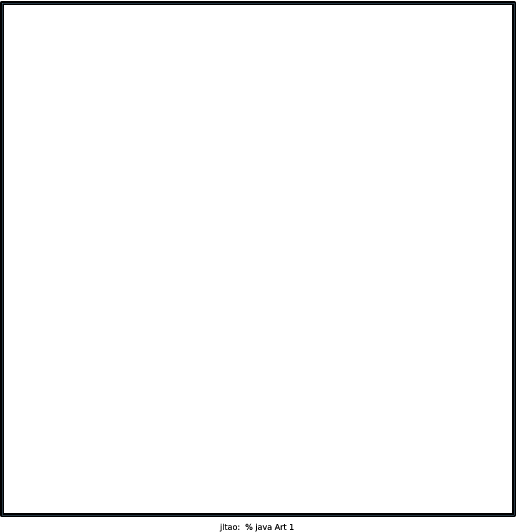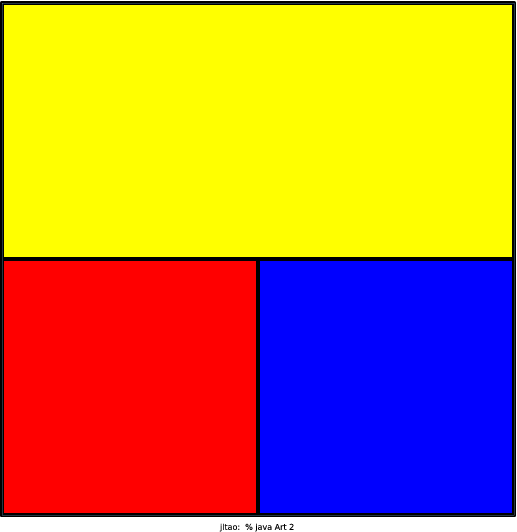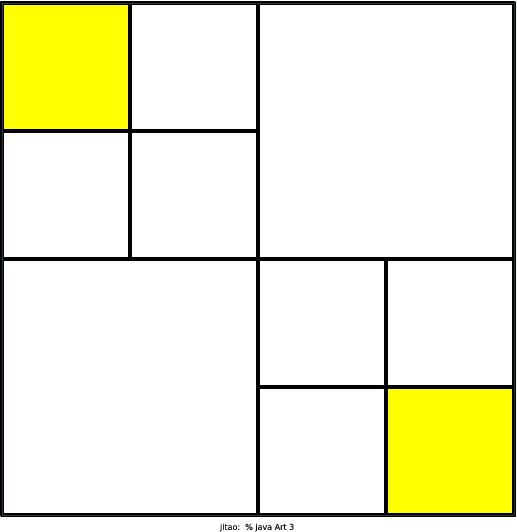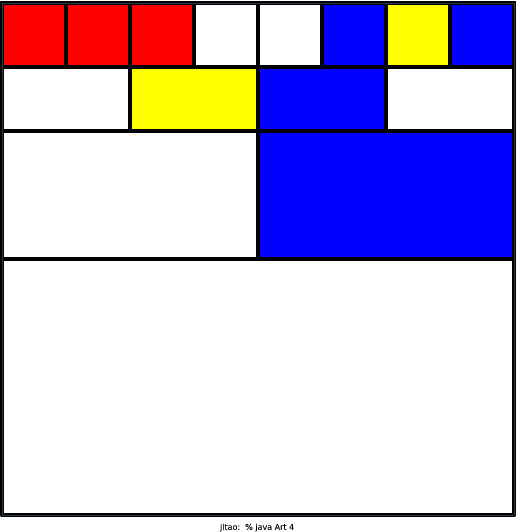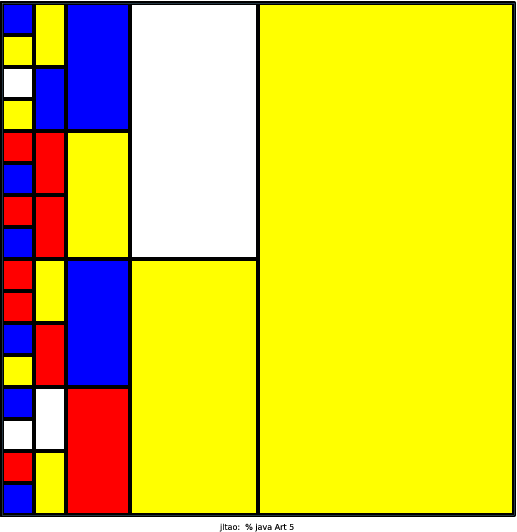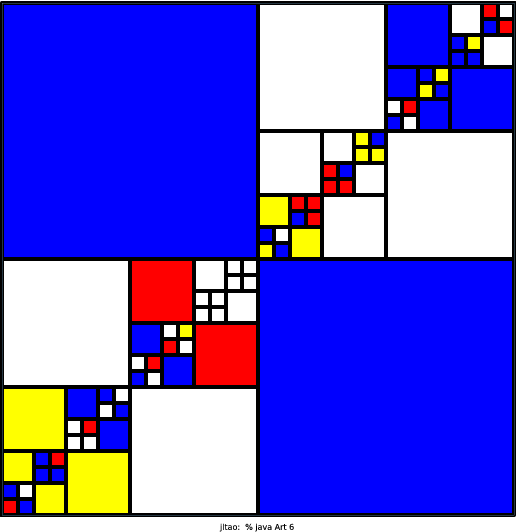Charlie Shen

I used the resources at Fractals Unleashed from the checklist and some wikipedia searches on fractals to choose what I would make. I made a program that creates a dragon curve starting from a single line. I found two ways to produce the curve. The first is to draw a line, then rotate it clockwise and draw it again (so you get an upside down L shape). You then take that shape and rotate it and draw it on top again (so you get kind of a backwards question mark). You continue to rotate the figure drawn and redraw it on top recursively, leading to the full dragon curve. As a result, each iteration of the curve has two elements of the previous iteration. The other way to do it, which is the method I used to program it, is to start with a single line segment. You rotate 45 degrees from this line and replace the original line with two segments of equal length, one along the 45 degree angle and the other perpendicular to the first. Essentially, you draw an isosceles right triangle pointing either clockwise or counter clockwise. From that point, you take the two segments you just drew and split them again, except that the second line is rotated the opposite direction. As a result, in my code, I recursive called two methods, one to rotate the first segment clockwise and the other to rotate the second segment counterclockwise. The actual line segment found is not drawn until the base case, because you replace the hypotenuse of the triangle each time.Kyle Xiao

Like Xiao Yang Yu and Samantha Ip (best of Spring 2015), I chose to make a program that would display a cherry blossom tree. I liked Xiao's idea more as it relied on randomness to generate a more organic picture. Rather than making a geometric shape that would be copied time after time, I chose to use a random number to determine the location of the next branch. Even though it's not a fractal, I think it's beautiful (especially when N is very large). All of the individual pink dots make the tree look very poised and elegant. Like Xiao, I chose to also scale the flowers and branches to help make the image look more organic. In essence, this is a reproduction of Xiao's program with a few minor repairs. I attempted to correct some of the issues that seemed to come up in his images. I made the flowers more flower shaped and increased the angle range for the branches.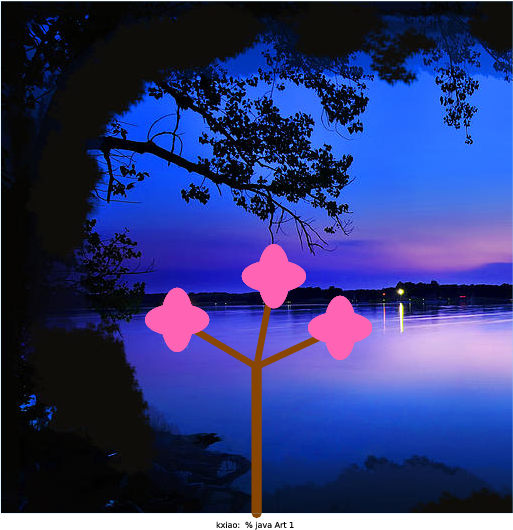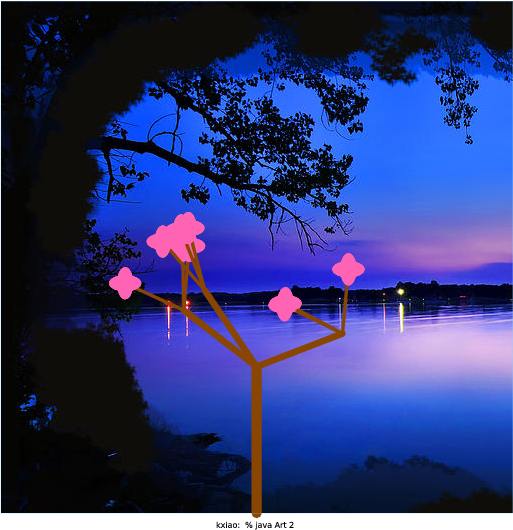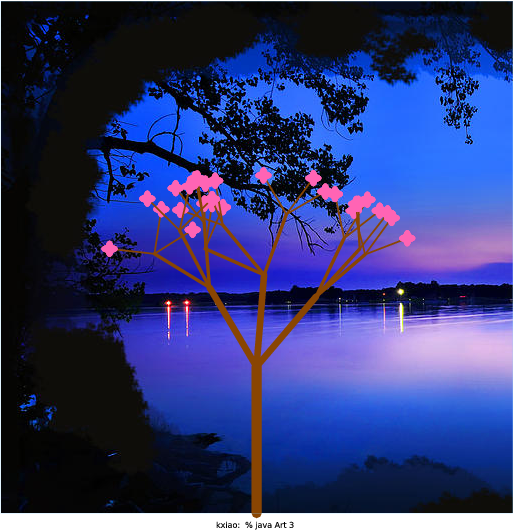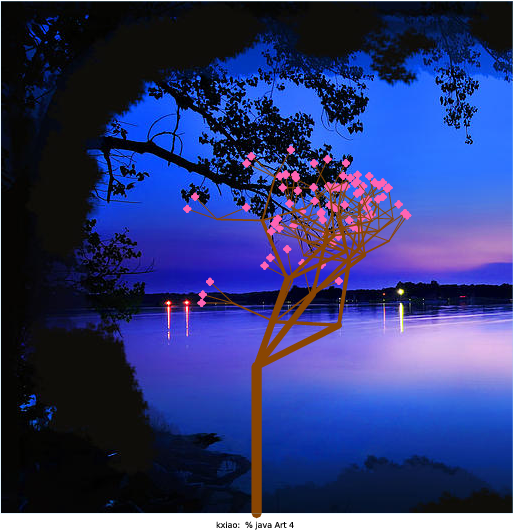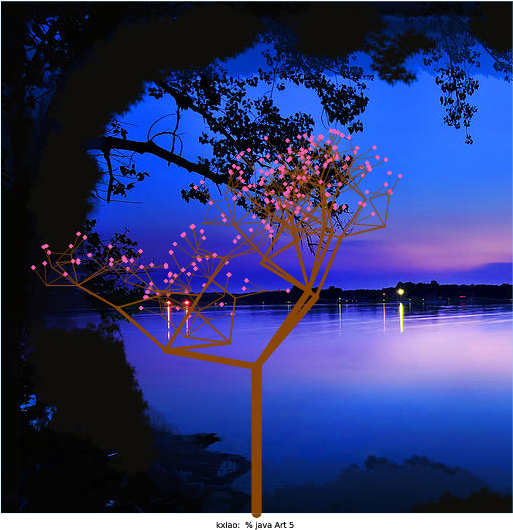Laura Herman

My program models de Stijl artist Piet Mondrian’s famous artworks. I used a recursive program that selects a random point at which to fracture the previous, larger rectangle into 4 smaller rectangles. I used another random function to fill in the rectangles with Mondrian’s famous color palette: bright red, yellow, and blue (while keeping white as the most likely option). Overall, I enjoyed the opportunity to mix abstract art with computer science.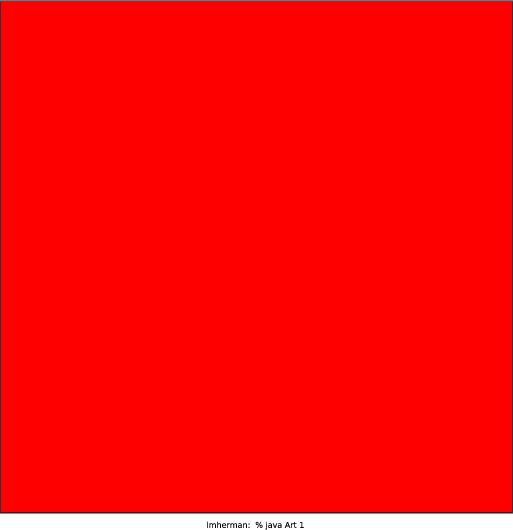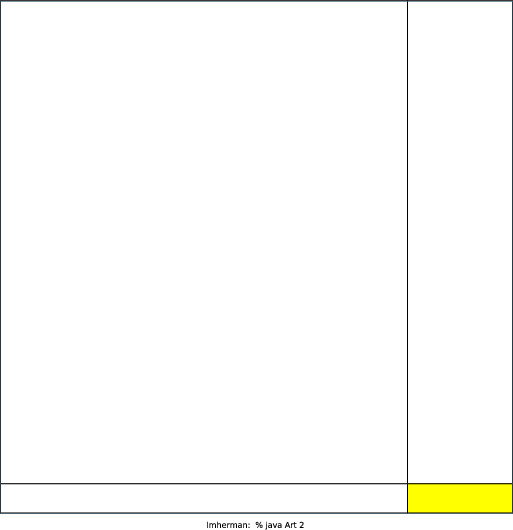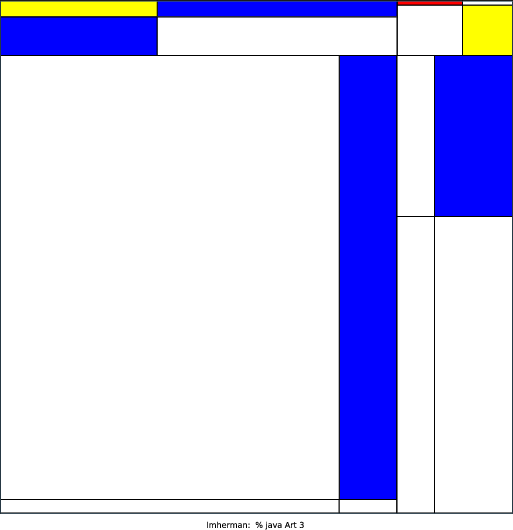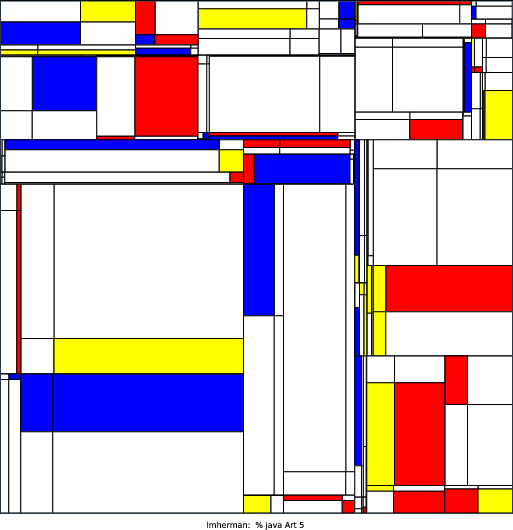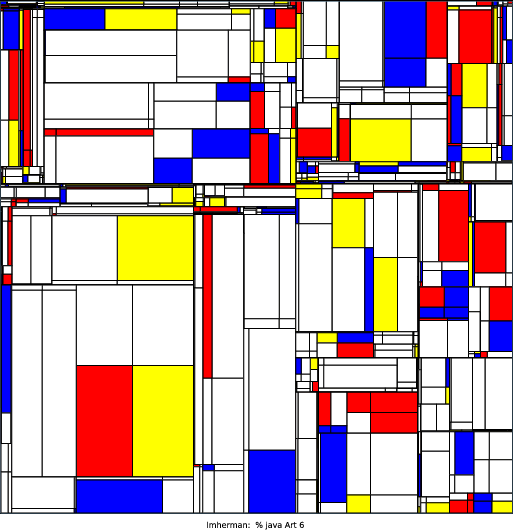Margaret Li

This program displays the Pythagoras Tree fractal, which I found out about from a link that the booksite recommended: http://wayback.archive-it.org/3635/20130719033956/http://library. thinkquest.org/26242/full/index.html I first started with the basic template from Sierpinski.java. I wrote some static class variables to hold constants, then had a main method, then wrote recursive method, and then had a draw method. I then wrote a draw method that drew one square at a specified angle. However, this didn’t work because the square on the left and the square on the right tilted in different directions. Apparently, the square on the right is basically the square on the left, rotated 360 - theta degrees, where theta is the tilt of the left square. So I rewrote the method drawSquares() to draw both squares at once. This involved lots of trig and bogged me down a lot. I ended up with lots of scribbled-over scratch paper. When I finally got the fractal to draw correctly, I decided to add some color to it.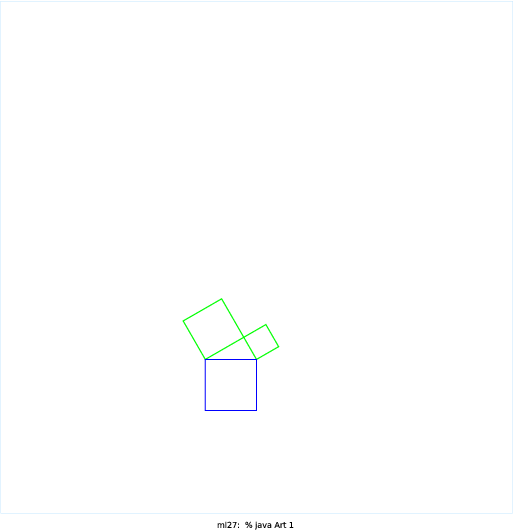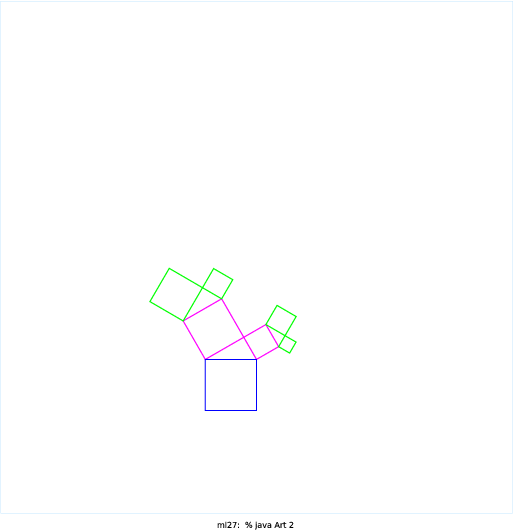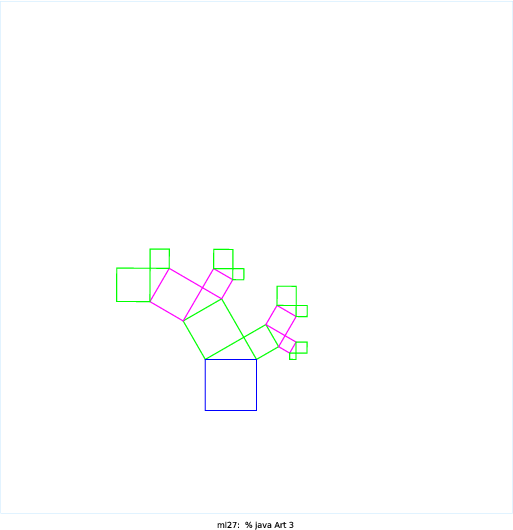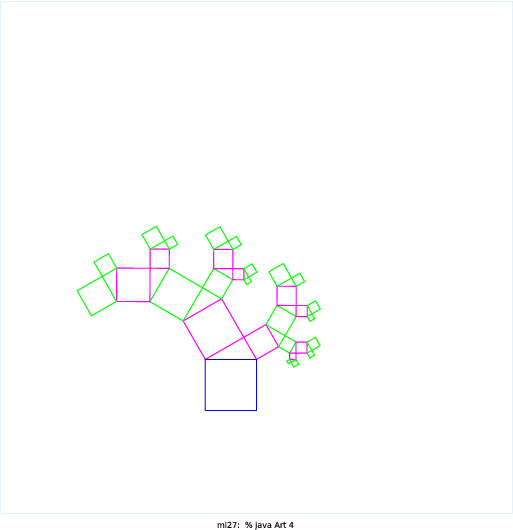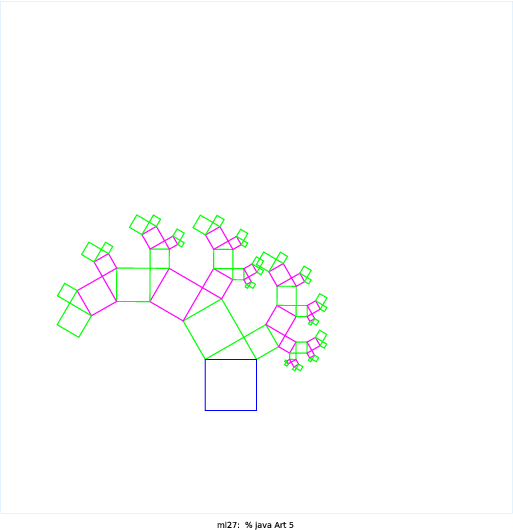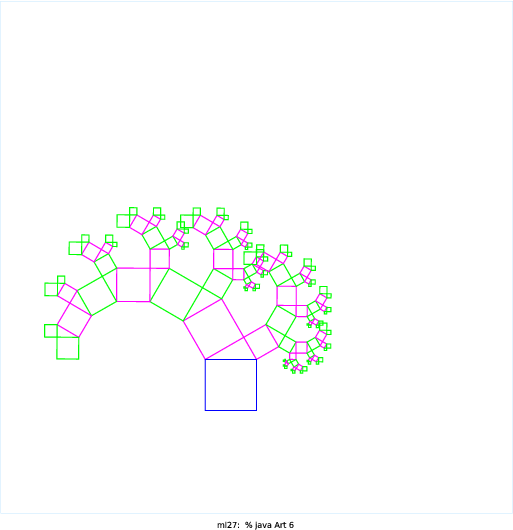My graphic recursively divides each cube into eight equal segments, and draws three of the cubes each time. The recursion also only occurs in the cubes that are re-drawn. The program utilizes colors so that each step may be viewed, and also gives the graphic a 3D effect.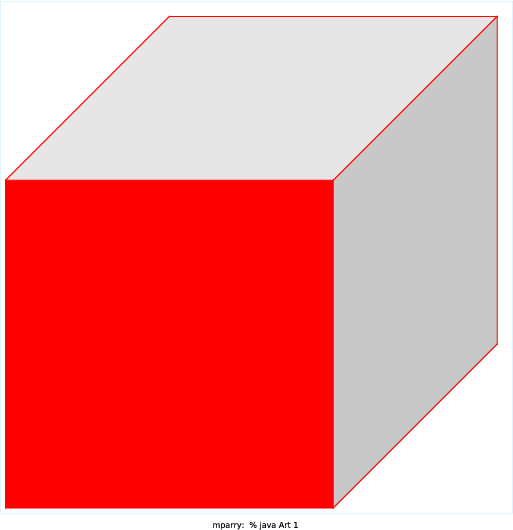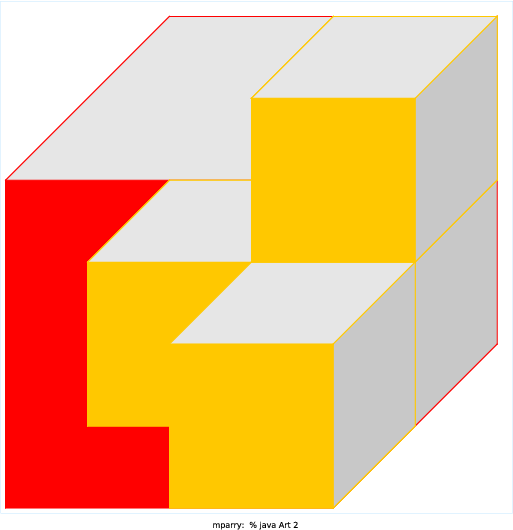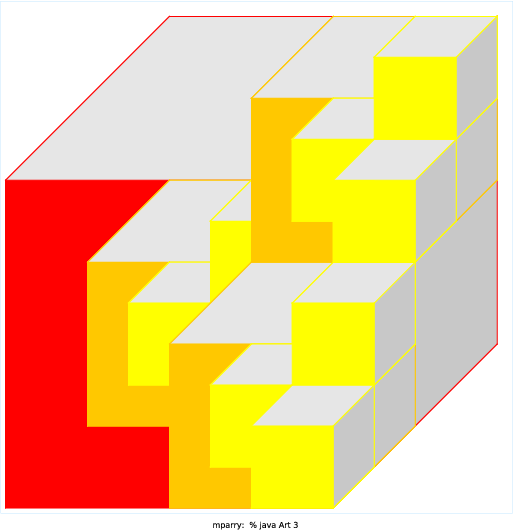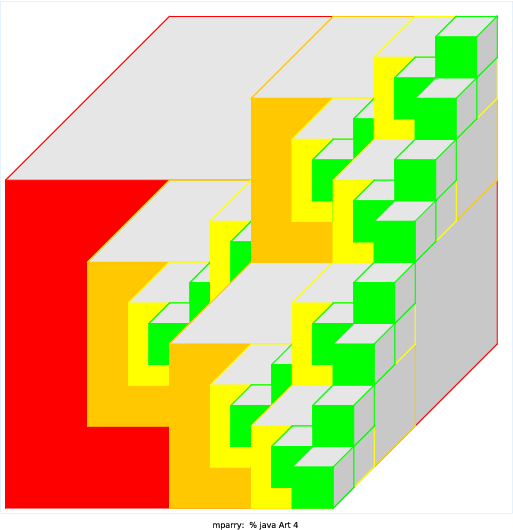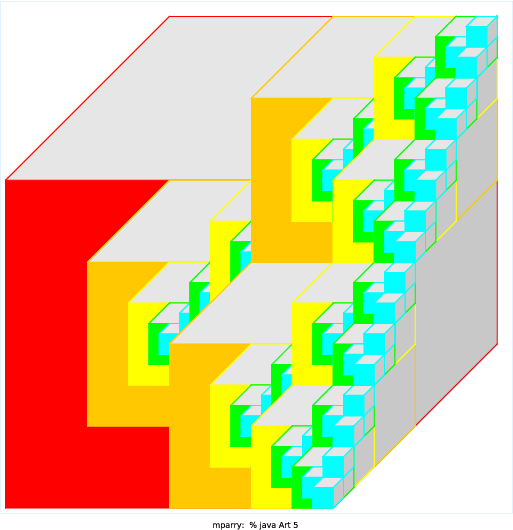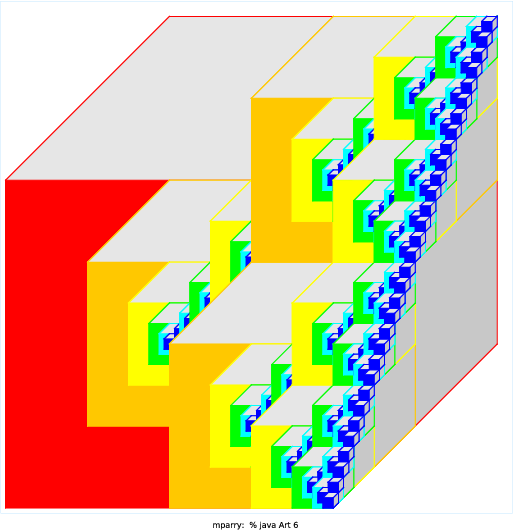Miki Somosot

I first looked up common fractals on Google images and found this recurring snowflake idea, but then when I was experimenting with the angles on my code (instead of using 45 degrees, I chose 30 degrees), I found that I liked this angle better because when you increase the number of branches/twigs, it mirrored the outer layer/outline of my favorite vegetable, broccoli.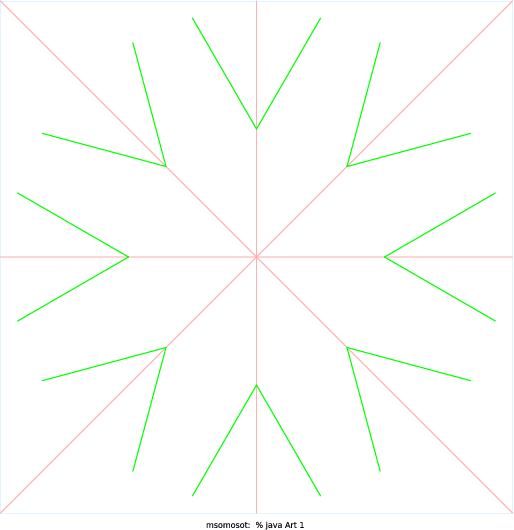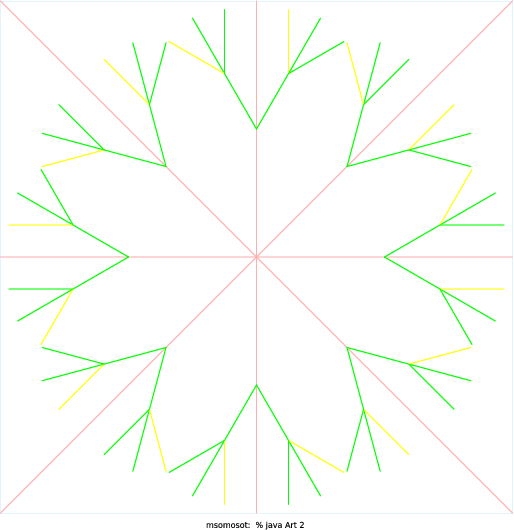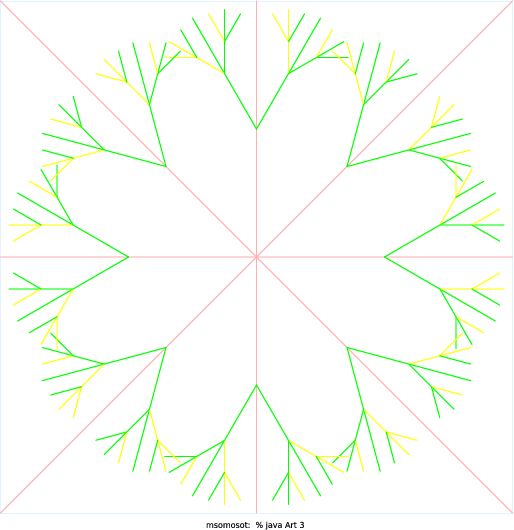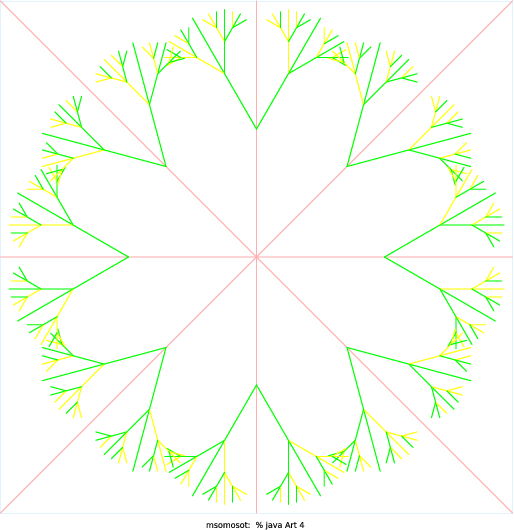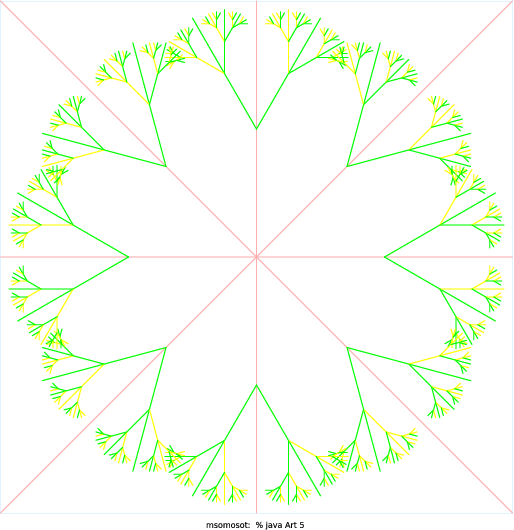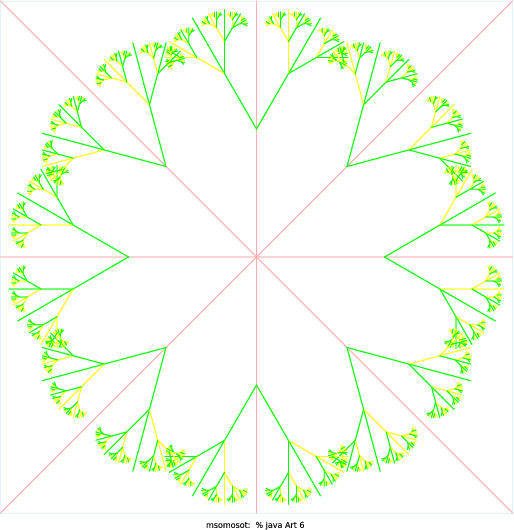Matt Tyler

I created a variation on the Apollonian Gasket, a fractal which I learned about at https://en.wikipedia.org/wiki/Apollonian_gasket. Instead of using three circles to start with, my program uses n + 2, where n is the first command-line argument (I add 2 because it works best with at least 3 circles initially). As a creativity augmenter, I colored each circle with an R, G and B value determined by its x-position, y-poition, and radius, respectively.